• 多功能ALU设计实验 一、实验目的与要求 实验目的： （1）学习多功能ALU的工作原理，掌握运算器的设计方法 （2）掌握运用Verilog HDL 进行行为描述与建模的技巧和方法 实验要求：本实验要求设计一个具有8种运算...
多功能ALU设计实验
一、实验目的与要求

实验目的：
（1）学习多功能ALU的工作原理，掌握运算器的设计方法
（2）掌握运用Verilog HDL 进行行为描述与建模的技巧和方法

实验要求：本实验要求设计一个具有8种运算功能的32位ALU，并能够产生运算结果的标志：结果为零标志ZF(Zero Flag)、溢出标志OF(Overflow Flag)。ALU通过3根控制线ALU_OP[2:0]来选择其8种功能。

功能表：

ALU_OP[2:0]
ALU_OP[2:0]
功能说明

0000
and
按位与运算

0001
or
按位或运

0010
xor
按位异或运算

0011
nor
按位或非运算

0100
add
算术加运算

0101
sub
算术减运算

0110
slt
若A<B，则输出1;否则输出0

0111
sll
B逻辑左移A所指定的位数

二、实验设计与程序代码
module ALU(OP,A,B,F,ZF,CF,OF,SF,PF);
parameter SIZE = 32;//运算位数
input [3:0] OP;//运算操作
input [SIZE:1] A;//左运算数
input [SIZE:1] B;//右运算数
output [SIZE:1] F;//运算结果
output  ZF, //0标志位, 运算结果为0(全零)则置1, 否则置0
CF, //进借位标志位, 取最高位进位C,加法时C=1则CF=1表示有进位,减法时C=0则CF=1表示有借位
OF, //溢出标志位，对有符号数运算有意义，溢出则OF=1，否则为0
SF, //符号标志位，与F的最高位相同
PF; //奇偶标志位，F有奇数个1，则PF=1，否则为0
reg [SIZE:1] F;
reg C,ZF,CF,OF,SF,PF;//C为最高位进位
always@(*)
begin
C=0;
case(OP)
4'b0000:begin F=A&B; end    //按位与
4'b0001:begin F=A|B; end    //按位或
4'b0010:begin F=A^B; end    //按位异或
4'b0011:begin F=~(A|B); end //按位或非
4'b0100:begin {C,F}=A+B; end //加法
4'b0101:begin {C,F}=A-B; end //减法
4'b0110:begin F=A<B; end    //A<B则F=1，否则F=0
4'b0111:begin F=B<<A; end   //将B左移A位
endcase
ZF = F==0;//F全为0，则ZF=1
CF = C; //进位借位标志
OF = A[SIZE]^B[SIZE]^F[SIZE]^C;//溢出标志公式
SF = F[SIZE];//符号标志,取F的最高位
PF = ~^F;//奇偶标志，F有奇数个1，则F=1；偶数个1，则F=0
end
endmodule


三、实验仿真
仿真代码👇
module Test();
reg [31:0] A,B;
reg [3:0] OP;
initial//初始化，每中运算擦拭两组数据
begin
/*按位与*/
OP=4'b0000;A=32'h0000_0000; B=32'h0000_0001;#50;
OP=4'b0000;A=32'h0000_0001; B=32'h0000_0001;#50;
/*按位或*/
OP=4'b0001;A=32'h0000_0000; B=32'h0000_0001;#50;
OP=4'b0001;A=32'h0000_0000; B=32'h0000_0000;#50;
/*按位异或*/
OP=4'b0010;A=32'h0000_0000; B=32'h0000_0001;#50;
OP=4'b0010;A=32'h0000_0000; B=32'h0000_0000;#50;
/*按位或非*/
OP=4'b0011;A=32'h0000_0000; B=32'h0000_0001;#50;
OP=4'b0011;A=32'h0000_0000; B=32'h0000_0000;#50;
/*算术加运算*/
OP=4'b0100;A=32'h7FFF_FFFF; B=32'h7FFF_FFFF;#50;
OP=4'b0100;A=32'hFFFF_FFFF; B=32'hFFFF_FFFF;#50;
/*算术减运算*/
OP=4'b0101;A=32'h7FFF_FFFF; B=32'h7FFF_FFFD;#50;
OP=4'b0101;A=32'h7FFF_FFFF; B=32'hFFFF_FFFF;#50;
/*A<B时,输出1，否则输出0*/
OP=4'b0110;A=32'h7FFF_FFFF; B=32'h8FFF_FFFF;#50;
OP=4'b0110;A=32'hFFFF_FFFF; B=32'h7FFF_FFFF;#50;
/*B逻辑左移A指定的位数*/
OP=4'b0111;A=32'h0000_0001; B=32'h0000_0001;#50;
OP=4'b0111;A=32'h0000_0001; B=32'h0000_0008;#50;
end
wire [31:0] F;
wire ZF, CF, OF, SF, PF;
ALU ALU_test(
.OP(OP),
.A(A),
.B(B),
.F(F),
.ZF(ZF),
.CF(CF),
.OF(OF),
.SF(SF),
.PF(PF)
);

endmodule


仿真波形👇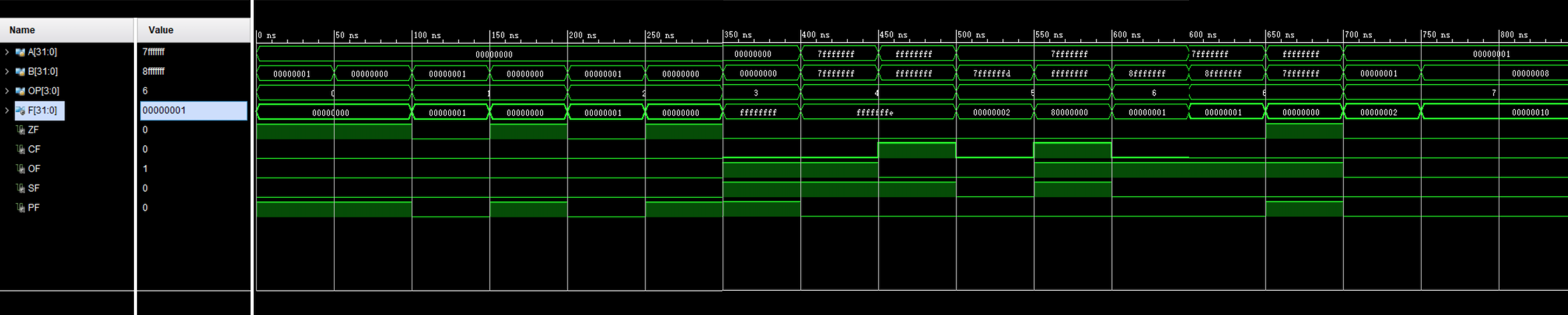四、电路图
展开全文• ALU设计，包含源码及仿真等内容，比较简单，仅供参考。
• 计算机组成原理上机报告，用Verilog语言实现多功能运算器ALU设计实验，仿真波形并书写实验报告。编程环境：Vivado HSL，设计语言：Verilog HDL。文档排版：LaTeX。内附实现代码，仿真波形截图，完整文档TeX源文件...
• 实验要求设计一个具有8种运算功能的32位ALU，并能够产生运算结果的表示：结果为零标志ZF(Zero Flag)、进借位标志位CF()溢出标志OF(Overflow Flag)、符号位标志SF（Sign Flag）和奇偶标志PF（Parity Flag）,标志位...
本实验要求设计一个具有8种运算功能的32位ALU，并能够产生运算结果的表示：结果为零标志ZF(Zero Flag)、进借位标志位CF()溢出标志OF(Overflow Flag)、符号位标志SF（Sign Flag）和奇偶标志PF（Parity Flag）,标志位值解释见代码。ALU通过4根控制线ALU_OP[2:0]来选择其8种功能，功能见表所示，多余的8位用于后继运算的拓展备用。
图中ALU_OP应该是三位，忽略最左侧的0程序代码
module ALU(
input [31:0]A,
input [31:0]B,
output reg [31:0]F,
output reg ZF,
output reg OF,
output reg SF,//符号标志
output reg PF,//奇偶标志
output reg CF,//进位/借位标志
input [2:0]ALU_OP
);
always @(*)
begin
OF=0;
CF=0;
case(ALU_OP)
3'b000://按位与
begin
F=A&B;
end
3'b001:F=A|B;//按位或
3'b010:F=A^B;//按位异或
3'b011://按位或非
begin
F=A|B;
F=~F;
end
3'b100://算术加
begin
{CF,F}=A+B;
OF=F^CF;
end
3'b101://算术减
begin
{CF,F}=A-B;
OF=F^CF;
CF=~CF;
end
3'b110://比较，A<B时出1，其他出0
begin
if(A<B) F=1;
else F=0;
end
3'b111:F=B<<A;//B逻辑左移A所指定的位数
endcase
end

always @(*)
begin
if(F==0) ZF=1;
else ZF=0;
end
always @(*)
begin
SF=F;
if(^F==0) PF=1;
else PF=0;
end

endmodule


测试代码
module ALU_test;

// Inputs
reg [31:0] A;
reg [31:0] B;
reg [2:0] ALU_OP;

// Outputs
wire [31:0] F;
wire ZF;
wire OF;
wire SF;
wire PF;
wire CF;

// Instantiate the Unit Under Test (UUT)
ALU uut (
.A(A),
.B(B),
.F(F),
.ZF(ZF),
.OF(OF),
.SF(SF),
.PF(PF),
.CF(CF),
.ALU_OP(ALU_OP)
);

initial begin
// Initialize Inputs
A = 0;
B = 0;
ALU_OP = 0;

// Wait 100 ns for global reset to finish
#10 A = 32'h0000_0010;B = 32'h0000_0011;ALU_OP = 3'b000;//按位与
#10 A = 32'h0000_0003;B = 32'h0000_0607;ALU_OP = 3'b001;//按位或
#10 A = 32'h8000_0000;B = 32'h8000_0001;ALU_OP = 3'b010;//按位异或
#10 A = 32'h7FFF_FFFF;B = 32'h7FFF_FFF1;ALU_OP = 3'b011;//按位或非
#10 A = 32'h3FFF_FFFF;B = 32'hFFFF_FFFF;ALU_OP = 3'b100;//算术加，有溢出
#10 A = 32'h0000_1010;B = 32'h0000_0001;ALU_OP = 3'b100;//算术加，无溢出
#10 A = 32'h8000_0000;B = 32'hFFFF_FFFF;ALU_OP = 3'b101;//算术减
#10 A = 32'hFFFF_FFFF;B = 32'h8000_0000;ALU_OP = 3'b110;//比较，A大
#10 A = 32'h8000_0000;B = 32'h8000_0000;ALU_OP = 3'b110;//比较，相等
#10 A = 32'h0000_0001;B = 32'h8000_0000;ALU_OP = 3'b110;//比较，A小
#10 A = 32'h1234_5678;B = 32'h3333_2222;ALU_OP = 3'b111;//B逻辑左移A位,全0
#10 A = 32'h0000_0001;B = 32'h3333_2222;ALU_OP = 3'b111;//B逻辑左移A位

// Add stimulus here

end

endmodule


仿真波形请各位自行设计实验项进行实验，这里就不放图了
软件采用ISE14.7


展开全文• 实验要求设计一个具有8种运算功能的32位ALU，并能够产生运算结果的表示：结果为零标志ZF(Zero Flag)和溢出标志OF(Overflow Flag)。ALU通过4根控制线ALU_OP[3:0]来选择其8种功能功能见表所示，多余的8位用于后继...
实验要求：        本实验要求设计一个具有8种运算功能的32位ALU，并能够产生运算结果的表示：结果为零标志ZF(Zero Flag)、进借位标志位CF()溢出标志OF(Overflow Flag)、符号位标志SF（Sign Flag）和奇偶标志PF（Parity Flag）,标志位值解释见代码。ALU通过4根控制线ALU_OP[3:0]来选择其8种功能，功能见表所示，多余的8位用于后继运算的拓展备用。功能表ALU_OP[3:0]ALU功能功能说明0000and按位与运算0001or按位或运算0010xor按位异或运算0011nor按位或非运算0100add算术加运算0101sub算术减运算0110slt若A<B，则输出1;否则输出00111sllB逻辑左移A所指定的位数实验IDE采用Vivado，开发语言Verilog。程序模块结构图：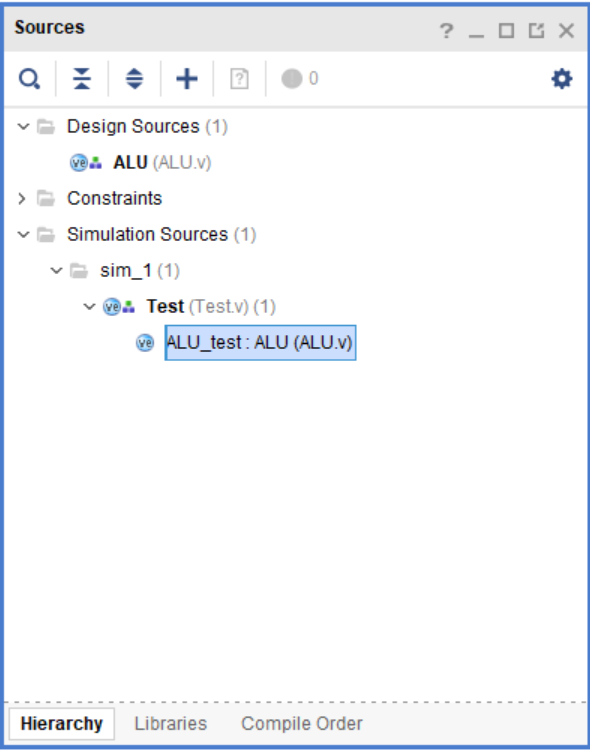ALU运算器模块代码：timescale 1ns / 1ps
//ALU模块
module ALU(OP,A,B,F,ZF,CF,OF,SF,PF);
parameter SIZE = 32;//运算位数
input [3:0] OP;//运算操作
input [SIZE:1] A;//左运算数
input [SIZE:1] B;//右运算数
output [SIZE:1] F;//运算结果
output  ZF, //0标志位, 运算结果为0(全零)则置1, 否则置0
CF, //进借位标志位, 取最高位进位C,加法时C=1则CF=1表示有进位,减法时C=0则CF=1表示有借位
OF, //溢出标志位，对有符号数运算有意义，溢出则OF=1，否则为0
SF, //符号标志位，与F的最高位相同
PF; //奇偶标志位，F有奇数个1，则PF=1，否则为0
reg [SIZE:1] F;
reg C,ZF,CF,OF,SF,PF;//C为最高位进位
always@(*)
begin
C=0;
case(OP)
4'b0000:begin F=A&B; end    //按位与
4'b0001:begin F=A|B; end    //按位或
4'b0010:begin F=A^B; end    //按位异或
4'b0011:begin F=~(A|B); end //按位或非
4'b0100:begin {C,F}=A+B; end //加法
4'b0101:begin {C,F}=A-B; end //减法
4'b0110:begin F=A<B; end    //A<B则F=1，否则F=0
4'b0111:begin F=B<<A; end   //将B左移A位
endcase
ZF = F==0;//F全为0，则ZF=1
CF = C; //进位借位标志
OF = A[SIZE]^B[SIZE]^F[SIZE]^C;//溢出标志公式
SF = F[SIZE];//符号标志,取F的最高位
PF = ~^F;//奇偶标志，F有奇数个1，则F=1；偶数个1，则F=0
end
endmodule
测试模块代码：timescale 1ns / 1ps
module Test();
reg [31:0] A,B;
reg [3:0] OP;
initial//初始化，每中运算擦拭两组数据
begin
/*按位与*/
OP=4'b0000;A=32'h0000_0000; B=32'h0000_0001;#50;
OP=4'b0000;A=32'h0000_0001; B=32'h0000_0001;#50;
/*按位或*/
OP=4'b0001;A=32'h0000_0000; B=32'h0000_0001;#50;
OP=4'b0001;A=32'h0000_0000; B=32'h0000_0000;#50;
/*按位异或*/
OP=4'b0010;A=32'h0000_0000; B=32'h0000_0001;#50;
OP=4'b0010;A=32'h0000_0000; B=32'h0000_0000;#50;
/*按位或非*/
OP=4'b0011;A=32'h0000_0000; B=32'h0000_0001;#50;
OP=4'b0011;A=32'h0000_0000; B=32'h0000_0000;#50;
/*算术加运算*/
OP=4'b0100;A=32'h7FFF_FFFF; B=32'h7FFF_FFFF;#50;
OP=4'b0100;A=32'hFFFF_FFFF; B=32'hFFFF_FFFF;#50;
/*算术减运算*/
OP=4'b0101;A=32'h7FFF_FFFF; B=32'h7FFF_FFFD;#50;
OP=4'b0101;A=32'h7FFF_FFFF; B=32'hFFFF_FFFF;#50;
/*A<B时,输出1，否则输出0*/
OP=4'b0110;A=32'h7FFF_FFFF; B=32'h8FFF_FFFF;#50;
OP=4'b0110;A=32'hFFFF_FFFF; B=32'h7FFF_FFFF;#50;
/*B逻辑左移A指定的位数*/
OP=4'b0111;A=32'h0000_0001; B=32'h0000_0001;#50;
OP=4'b0111;A=32'h0000_0001; B=32'h0000_0008;#50;
end
wire [31:0] F;
wire ZF, CF, OF, SF, PF;
ALU ALU_test(
.OP(OP),
.A(A),
.B(B),
.F(F),
.ZF(ZF),
.CF(CF),
.OF(OF),
.SF(SF),
.PF(PF)
);

endmodule
仿真波形图：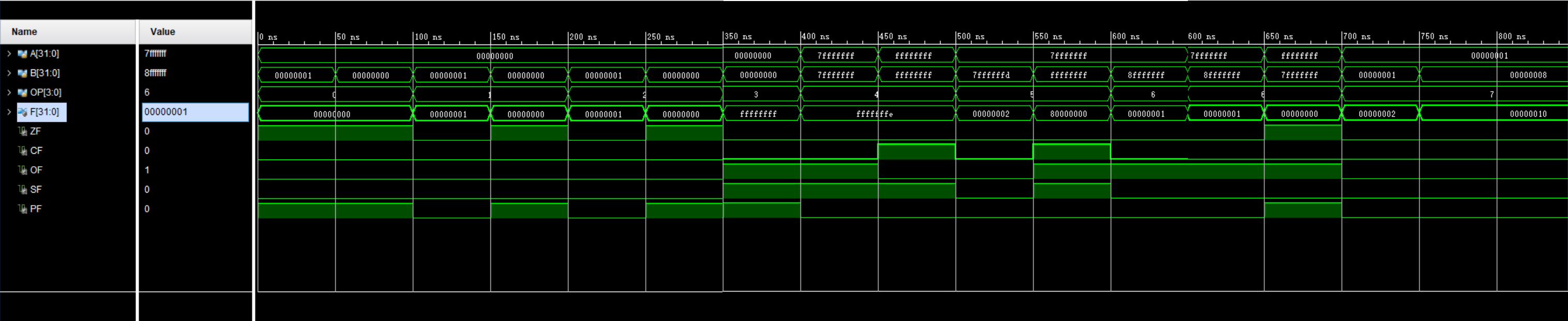仿真电路图：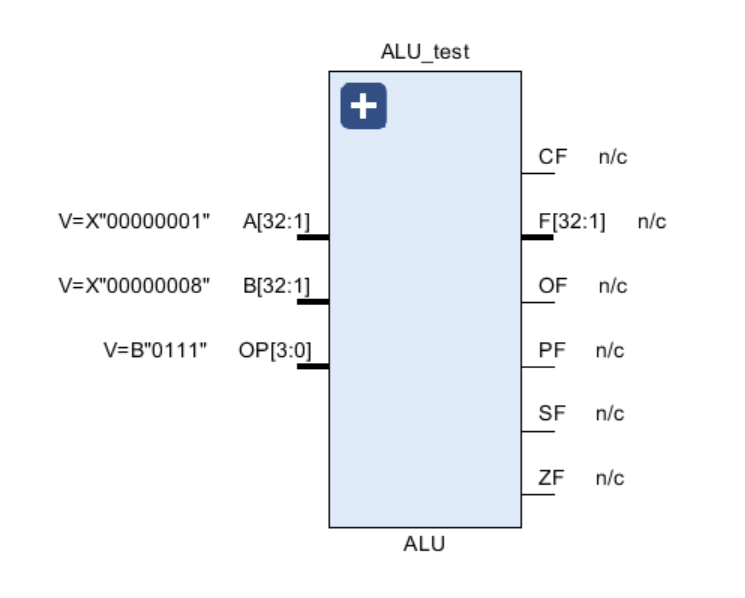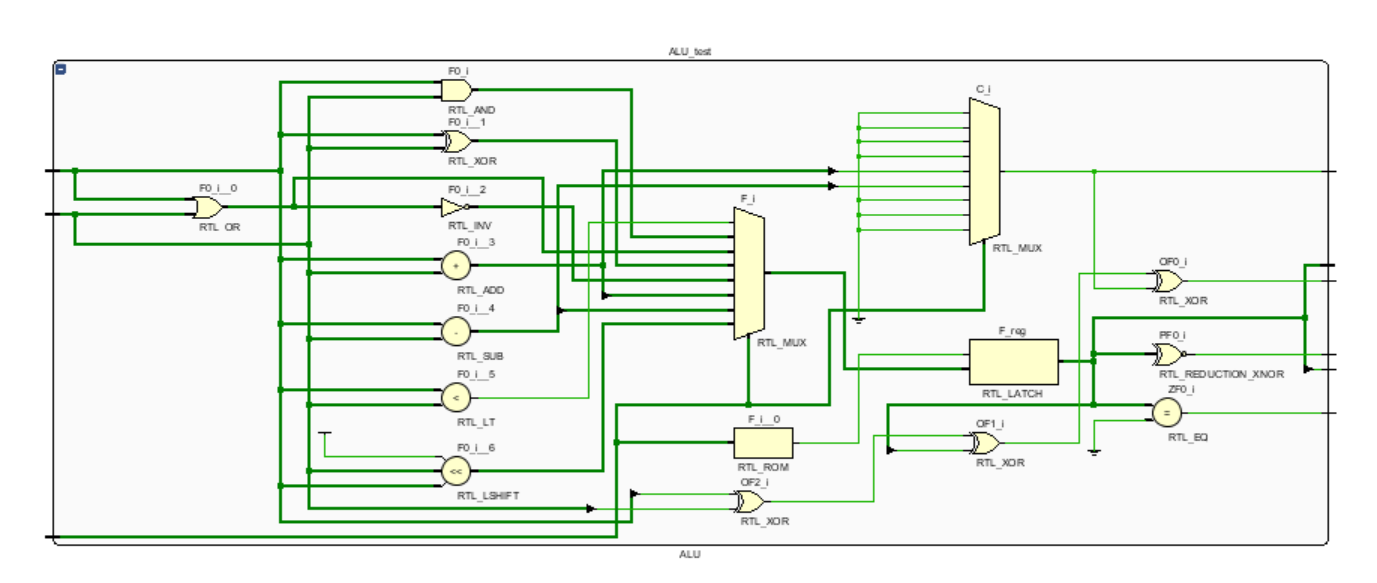心得体会：    总的来说实验三不是很难吧，本来书上的要求是做OP[2:0]三位8中运算，标志位也没有那么多。章老师开了翻转课堂，给我们加了一些要求。这是本学期我们的第一个实验，很多Verilog的语法都忘记了，磕磕碰碰和组员讨论，debug。非常感谢另外两个小伙伴lym和tk。
展开全文计算机组成原理 ALU运算器
• 1. 学习多功能ALU的工作原理，掌握运算器的设计方法 2. 掌握运用Verilog HDL 进行行为描述与建模的技巧和方法 解决方法 1. 分析一个具有8种运算功能的32位ALU，并能够产生运算结果的标志：结果位零标志（ZF）和溢出...
实验内容
1.	学习多功能ALU的工作原理，掌握运算器的设计方法
2.	掌握运用Verilog HDL 进行行为描述与建模的技巧和方法
解决方法
1.	分析一个具有8种运算功能的32位ALU，并能够产生运算结果的标志：结果位零标志（ZF）和溢出标志（OF）
2.	首先，分析出ZF只会在加减运算中出现溢出情况，然后分析出ALU 8种计算如何表达，如果使用“-”，就不用考虑如何减法
3.	代码展示：
顶层模块：
module ALU(AB_SW,ALU_OP,F_LED_SW,LED);
input[2:0]AB_SW,ALU_OP,F_LED_SW;
output[7:0]LED;
wire [31:0]A,B,F;
wire ZF,OF;
Third_experiment_second T2(AB_SW,A,B);
Third_experiment_first T1(OF,ZF,ALU_OP,A,B,F);
Third_experiment_third T3(F_LED_SW,LED,F,ZF,OF);

endmodule

Third_experiment_second模块（作为数据输入）
module Third_experiment_second(AB_SW,A,B);
input[2:0]AB_SW;
output reg[31:0]A,B;
always @(*)
begin
case(AB_SW)
3'b000:begin A=32'h0000_0000;B=32'h0000_0000;end
3'b001:begin A=32'h0000_0003;B=32'h0000_0607;end
3'b010:begin A=32'h8000_0000;B=32'h8000_0000;end
3'b011:begin A=32'h7FFF_FFFF;B=32'h7FFF_FFFF;end
3'b100:begin A=32'hFFFF_FFFF;B=32'hFFFF_FFFF;end
3'b101:begin A=32'h8000_0000;B=32'hFFFF_FFFF;end
3'b110:begin A=32'hFFFF_FFFF;B=32'h8000_0000;end
3'b111:begin A=32'h1234_5678;B=32'h3333_2222;end
default:begin A=32'h9ABC_DEF0;B=32'h1111_2222;end
endcase
end
endmodule

Third_experiment_first模块（运算）
module Third_experiment_first(OF,ZF,ALU_OP,A,B,F);
input [2:0]ALU_OP;
input [31:0]A,B;
output reg[31:0]F;
reg C32;
output reg OF=0;
output reg ZF=0;
always @(ALU_OP or A or B)
begin
case(ALU_OP)
3'b000:F<=A&B;
3'b001:F<=A|B;
3'b010:F<=A^B;
3'b011:F<=A~^B;
3'b100:{C32,F}<=A+B;
3'b101:{C32,F}<=A-B;
3'b110:begin if(A<B)  F<=32'h0000_0001;else F<=32'h0000_0000;end
3'b111:begin F<=B<<A;end
endcase
if(F==32'h0000_0000)
ZF<=1;
else
ZF<=0;
OF=C32^F^A^B;
end
endmodule

Third_experiment_third模块（作为LED显示）
module Third_experiment_third(F_LED_SW,LED,F,ZF,OF);
input[31:0]F;
input ZF,OF;
input[2:0] F_LED_SW;
output reg[7:0] LED;
always@(*)
begin
case(F_LED_SW)
3'b000:LED=F[7:0];
3'b001:LED=F[15:8];
3'b010:LED=F[23:16];
3'b011:LED=F[31:24];
default:begin LED=ZF;LED=OF;LED[6:1]=6'b0;end
endcase
end

endmodule


测试模块
module ALU_TEST;
// Inputs
reg [2:0] AB_SW;
reg [2:0] ALU_OP;
reg [2:0] F_LED_SW;
// Outputs
wire [7:0] LED;
ALU uut (
.AB_SW(AB_SW),
.ALU_OP(ALU_OP),
.F_LED_SW(F_LED_SW),
.LED(LED)
);
initial begin
AB_SW = 3'b001;
ALU_OP = 3'b000;
F_LED_SW = 3'b000;
#100;
AB_SW = 3'b001;
ALU_OP = 3'b001;
F_LED_SW = 3'b000;
#100;
AB_SW = 3'b001;
ALU_OP = 3'b010;
F_LED_SW = 3'b000;
end
endmodule

特别注意
当时在做实验的时候，有的朋友问我begin…end是干什么用，其实就是相当于C语言中的大括号


展开全文• 多功能ALU运算器设计实验实验内容模块说明ALU模块代码（带CF,SF,PF版本）ALU模块代码（不带CF,SF,PF版本）测试用例代码逻辑引脚图仿真截图思考与探索 实验内容   本实验要求设计一个具有16种运算功能的32位ALU，并...
• 杭电计算机组成实验3（三）多功能ALU设计实验 杭电计算机组成实验4（四）寄存器堆设计实验 杭电计算机组成实验5（五）存储器设计实验 杭电计算机组成实验6（六）MIPS汇编器与模拟器实验 杭电计算机组成实验7（七）取...
• 实现R型指令的CPU设计实验实验内容实验内容与原理汇编指令与.coe文件功能模块说明逻辑引脚图仿真时序波形图R-I CPU 完整代码测试用例代码探索与思考（非标准答案） 实验内容 选以下4种系统结构之一，设计一个MIPS单...
• 需要完成的功能是 Mem[PC++] → IR 计算+1的动作和访问内存可以并行 但是内存取出来的地址和ALU的结果必须分不同的周期送回 节拍 数据通路 控制信号 说明 T1 Mem[PC]→IR,(PC)→AluSrcA,(AluOut)→PC IRin,...
• ## 多周期CPU实验

千次阅读 2017-07-09 08:35:41
1.数据通路图 与单周期CPU设计相比，图上增加IR指令寄存器，目的是使指令代码保持稳定，pc写使能控制信号PCWre，是确保pc适时修改，原因都是和周期工作的CPU有关。...3.ALU运算功能表4.实验内容 5.cpu
• 学生掌握一位全加器的实现逻辑，掌握位可控加减法电路的实现逻辑，熟悉 Logisim 平台基本功能，能在 logisim 中实现位可控加减法电路。 实验内容 在 logisim 模拟器中打开 alu.circ 文件，在对应子电路中利用...
• 实验一 运算器实验实验二 移位运算实验实验三 存储器读写和总线控制实验附加实验 总线控制实验实验五 微程序设计实验 一、实验目的： 1. 掌握运算器的组成及工作原理； 2. 了解4位函数发生器74LS181的组合功能，...
• 1、 设计一个“10010”的序列监测器 ...1、 用函数设计一个实现8位ALU功能的函数，并熟悉条件语句，分支语句的应用。 2、 设计它的激励模块，选择适当的激励条件来验证激励。 3、 进一步熟悉ModelSim软件的功能
• Cache实验中，cache容量大？区表存储器是用来做什么的？ Cache失效时，如何进行操作？ 按钮4次，3次会产生什么情况？ 总线实验中，K6、k7、E0-E3的作用是什么？ 总线是如何实现清中断操作的？如何判断已经锁死外部...
• 8 ALU设计 请给出ALU的VHDL功能描述。将其作为QuartusII的输入文件，由QuartusII进行综合和模拟，自己设计测试向量波形，并通过分析模拟结果验证设计的正确性。 选做 设计 专业基础 计算机组成原理实验箱60台 P4...
• 实验设计的RISC_CPU由图1所示ALU、CU、ACC、IR、PC等个单元电路组成，配合程序存储器ROM及数据存储器RAM实现程序运行功能。 图1实验电路结构图 2.设计步骤 基于图1设计8条指令，指令格式为： OP（ 3...cpu
• 帮助学生掌握一位全加器的实现逻辑，掌握位可控加减法电路的实现逻辑，熟悉 Logisim 平台基本功能，能在 logisim 中实现位可控加减法电路。 实验内容 在 Logisim 模拟器中打开 alu.circ 文件，在对应子电路中...
• 学生掌握一位全加器的实现逻辑，掌握位可控加减法电路的实现逻辑，熟悉 Logisim 平台基本功能，能在 logisim 中实现位可控加减法电路。 实验内容 在 logisim 模拟器中打开 alu.circ 文件，在对应子电路中利用...